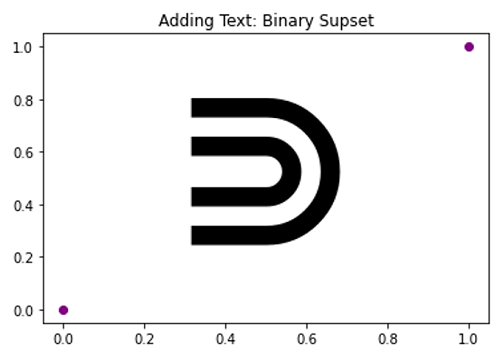# Binary Supset Symbol in Python Plotting

Here, we are going to learn how to add binary supset symbol in Python plotting?
Submitted by Anuj Singh, on September 15, 2020

Binary Supset a different symbol used in set theory and other mathematical expressions. Python has provided us to use it while plotting.

Following example shows its implementation.

```plt.text(0.25, 0.25, r'\$\Supset\$', fontsize=175)
```

Illustrations:## Python code for Binary Supset symbol in plotting

```import numpy as np
import matplotlib.pyplot as plt

# Binary Supset
# In text
plt.figure()
plt.plot([0,1],[0,1], 'o', color=  'purple')
plt.text(0.25, 0.25, r'\$\Supset\$', fontsize=175, )
plt.show()

plt.figure()
plt.plot([0,1],[0,1], 'o', color=  'purple')
plt.text(0.25, 0.25, r'\$\Supset\$', fontsize=175, )
plt.axis(False)
plt.show()
```

Output:

```Output is as Figure
```

Languages: » C » C++ » C++ STL » Java » Data Structure » C#.Net » Android » Kotlin » SQL
Web Technologies: » PHP » Python » JavaScript » CSS » Ajax » Node.js » Web programming/HTML
Solved programs: » C » C++ » DS » Java » C#
Aptitude que. & ans.: » C » C++ » Java » DBMS
Interview que. & ans.: » C » Embedded C » Java » SEO » HR
CS Subjects: » CS Basics » O.S. » Networks » DBMS » Embedded Systems » Cloud Computing
» Machine learning » CS Organizations » Linux » DOS
More: » Articles » Puzzles » News/Updates